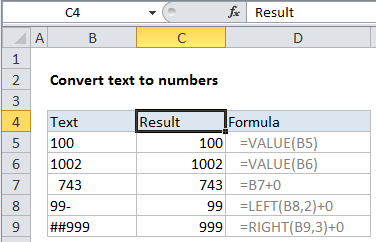# Convert text to numbers using VALUE function in Excel

To convert simple text values to numbers, you can use the the VALUE function, or simply add zero as described below.

## Formula

`=VALUE(A1)`## Explanation

In the example shown, the formula in C5 is:

`=VALUE(B5)`

### Background

Sometimes Excel ends up with text in a cell, when you really want a number. There are many reasons this might happen, and many ways to fix. This article describes a formula-based approach convert text values to numbers.

Worked Example:   How to create email address with name and domain in Excel

### How this formula works

In this example, the values in column A are “stored as text”. This means if you try to SUM column A, you’ll get a result of zero.

The VALUE function will try to “coerce” a number stored as text to a true number. In simple cases, it will just work and you’ll get a numeric result. If it doesn’t work, you’ll get a #VALUE error.  Visit Convert Text to Numbers to see other examples.

Worked Example:   Convert column number to letter in Excel

Another common trick is to simply add zero to the text value to convert. This forces Excel to try and convert the text value to a number to handle the math operation. This has the same functionality as VALUE. Cell C7 uses this formula.

### Stripping other characters

If a cell contains non-numeric characters like dashes, punctuation, and so on, you’ll need to apply more effort.

Worked Example:   How to get Excel workbook path only

The formulas in C8 and C9 show how to use the LEFT and RIGHT functions to strip non-numeric characters from a value before it’s converted to a number. You can also use the MID function in more complicated situations.

If you need to strip extra spaces or other non-printing characters, see the TRIM and CLEAN functions.

Finally, the SUBSTITUTE function will let you strip other characters that appear in random locations inside a value.